# Mass Force and GravityPage 1

#### WATCH ALL SLIDES

Slide 1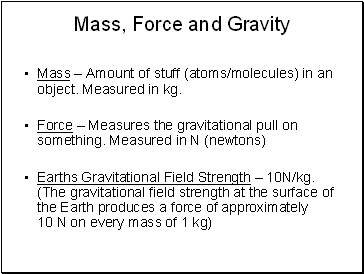## Mass, Force and Gravity

Mass – Amount of stuff (atoms/molecules) in an object. Measured in kg.

Force – Measures the gravitational pull on something. Measured in N (newtons)

Earths Gravitational Field Strength – 10N/kg. (The gravitational field strength at the surface of the Earth produces a force of approximately 10 N on every mass of 1 kg)

Slide 2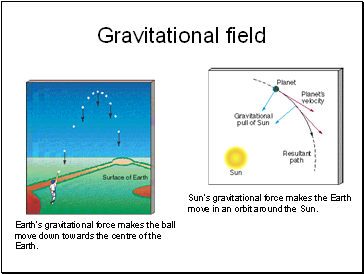## Gravitational field

Earth’s gravitational force makes the ball move down towards the centre of the Earth.

Sun’s gravitational force makes the Earth move in an orbit around the Sun.

Slide 3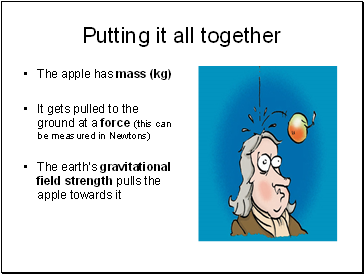## Putting it all together

The apple has mass (kg)

It gets pulled to the ground at a force (this can be measured in Newtons)

The earth’s gravitational field strength pulls the apple towards it

Slide 4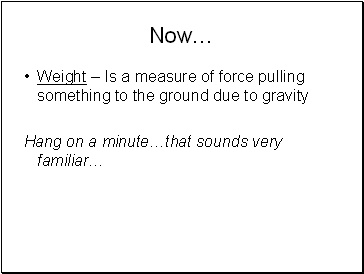Now…

Weight – Is a measure of force pulling something to the ground due to gravity

Hang on a minute…that sounds very familiar…

Slide 5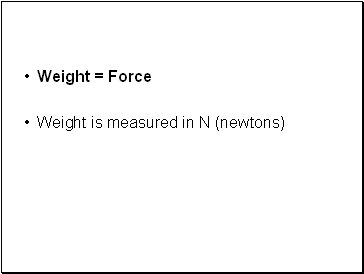Weight = Force

Weight is measured in N (newtons)

Slide 6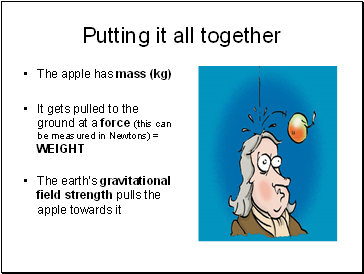## Putting it all together

The apple has mass (kg)

It gets pulled to the ground at a force (this can be measured in Newtons) = WEIGHT

The earth’s gravitational field strength pulls the apple towards it

Slide 7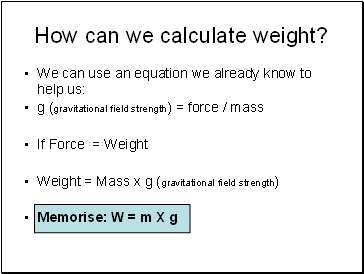## How can we calculate weight?

We can use an equation we already know to help us:

g (gravitational field strength) = force / mass

If Force = Weight

Weight = Mass x g (gravitational field strength)

Memorise: W = m X g